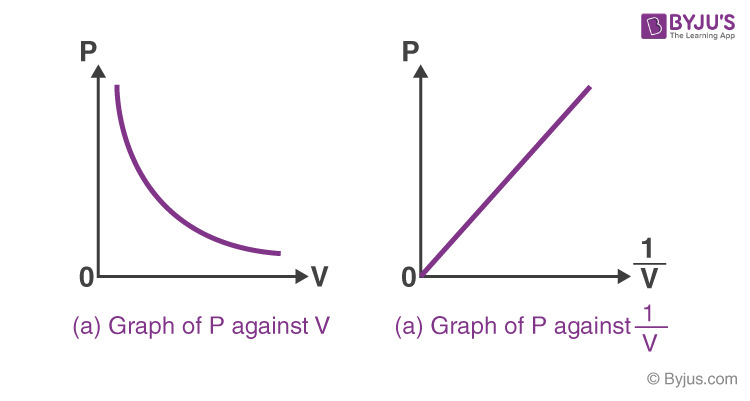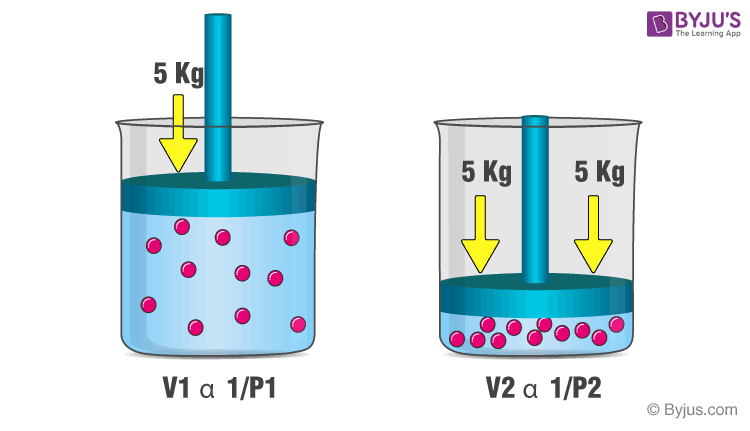Checkout JEE MAINS 2022 Question Paper Analysis : Checkout JEE MAINS 2022 Question Paper Analysis :

# Boyle’s Law

## What is Boyle’s Law?

Boyle’s law is a gas law which states that the pressure exerted by a gas (of a given mass, kept at a constant temperature) is inversely proportional to the volume occupied by it. In other words, the pressure and volume of a gas are inversely proportional to each other as long as the temperature and the quantity of gas are kept constant. Boyle’s law was put forward by the Anglo-Irish chemist Robert Boyle in the year 1662.

## Recommended VideosFor a gas, the relationship between volume and pressure (at constant mass and temperature) can be expressed mathematically as follows.

P ∝ (1/V)

Where P is the pressure exerted by the gas and V is the volume occupied by it. This proportionality can be converted into an equation by adding a constant, k.

P = k*(1/V) ⇒ PV = k

The pressure v/s volume curve for a fixed amount of gas kept at constant temperature is illustrated below.Boyle’s law

It can be observed that a straight line is obtained when the pressure exerted by the gas (P) is taken on the Y-axis and the inverse of the volume occupied by the gas (1/V) is taken on the X-axis.

## Formula and Derivation

As per Boyle’s law, any change in the volume occupied by a gas (at constant quantity and temperature) will result in a change in the pressure exerted by it. In other words, the product of the initial pressure and the initial volume of a gas is equal to the product of its final pressure and final volume (at constant temperature and number of moles). This law can be expressed mathematically as follows:

P1V1 = P2V2

Where,

• P1 is the initial pressure exerted by the gas
• V1 is the initial volume occupied by the gas
• P2 is the final pressure exerted by the gas
• V2 is the final volume occupied by the gas

This expression can be obtained from the pressure-volume relationship suggested by Boyle’s law. For a fixed amount of gas kept at a constant temperature, PV = k. Therefore,

P1V1 = k (initial pressure * initial volume)

P2V2 = k (final pressure * final volume)

∴ P1V1 = P2V2

This equation can be used to predict the increase in the pressure exerted by a gas on the walls of its container when the volume of its container is decreased (and its quantity and absolute temperature remain unchanged).

## Examples of Boyle’s Law

When a filled balloon is squeezed, the volume occupied by the air inside the balloon decreases. This is accompanied by an increase in the pressure exerted by the air on the balloon, as a consequence of Boyle’s law. As the balloon is squeezed further, the increasing pressure eventually pops it. An illustration describing the increase in pressure that accompanies a decrease in the volume of a gas is provided below.Examples of Boyle’s Law

If a scuba diver rapidly ascends from a deep zone towards the surface of the water, the decrease in the pressure can cause the gas molecules in his/her body to expand. These gas bubbles can go on to cause damage to the diver’s organs and can also result in death. This expansion of the gas caused by the ascension of the scuba diver is another example of Boyle’s law. Another similar example can be observed in the deep-sea fish that die after reaching the surface of the water (due to the expansion of dissolved gasses in their blood).

## Solved Exercises on Boyle’s Law

### Exercise 1

A fixed amount of a gas occupies a volume of 1L and exerts a pressure of 400 kPa on the walls of its container. What would be the pressure exerted by the gas if it is completely transferred into a new container having a volume of 3 liters (assuming the temperature and quantity of gas remains constant)?

Given,

Initial volume (V1) = 1L

Initial pressure (P1) = 400 kPa

Final volume (V2) = 3L

As per Boyle’s law, P1V1 = P2V2 ⇒ P2 = (P1V1)/V2

P2 = (1L * 400 kPa)/3L = 133.33 kPa

Therefore, the gas exerts a pressure of 133.33 kPa on the walls of the 3-liter container.

### Exercise 2

A gas exerts a pressure of 3 kPa on the walls of container 1. When container 1 is emptied into a 10-liter container, the pressure exerted by the gas increases to 6 kPa. Find the volume of container 1. Assume that the temperature and quantity of the gas remain constant.

Given,

Initial pressure, P1 = 3kPa

Final pressure, P2 = 6kPa

Final volume, V2 = 10L

According to Boyle’s law, V1 = (P2V2)/P1

V1 = (6 kPa * 10 L)/3 kPa = 20 L

Therefore, the volume of container 1 is 20 L.

## Frequently Asked Questions – FAQs

### How does Boyle’s law work?

Boyle’s law is a gas law that states that a gas’s pressure and volume are inversely proportional. When the temperature is kept constant, as volume increases, pressure falls and vice versa.

### Why is Boyle law important?

Boyle’s law is significant because it explains how gases behave. It proves beyond a shadow of a doubt that gas pressure and volume are inversely proportional. When you apply pressure on a gas, the volume shrinks and the pressure rises.

### What is the formula for Boyle’s gas law?

The empirical relation asserts that the pressure (p) of a given quantity of gas changes inversely with its volume (v) at constant temperature; i.e., pv = k, a constant, as proposed by physicist Robert Boyle in 1662.

### What is a good example of Boyle’s Law?

A balloon is a good example of Boyle’s law in action. The balloon is inflated by blowing air into it; the pressure of the air pulls on the rubber, causing the balloon to expand. When one end of the balloon is compressed, the pressure within rises, causing the un-squeezed section of the balloon to expand outward.

### Can Boyle’s law be experimentally proven?

Boyle’s law is a connection between pressure and volume. It asserts that under constant temperature, the pressure of a specific quantity of gas is inversely proportional to its volume. It is possible to prove the law empirically. The paper discusses a syringe-based experimental approach for verifying the law.

### What is Boyle’s law?

Boyle’s law is a gas law given by the Anglo-Irish chemist Robert Boyle in 1662. He stated that the pressure exerted by a gas is inversely proportional to the volume occupied by it at a constant mass and temperature.

### What is the relationship between pressure and volume?

The pressure and volume are inversely proportional to each other under Boyle’s law.
P ∝ (1/V)

### Why does volume decrease when pressure is increased?

Volume decreases with increasing pressure because the gas particles come close to each other with increasing pressure. Similarly, volume increases with decreasing pressure because the gas particles go far away from each other with decreasing pressure.

### What happens to pressure if the volume is doubled?

For a fixed mass of gas at a constant temperature, pressure is inversely proportional to volume. If the volume is doubled, the pressure will be halved.

### Why Boyle’s law is not applicable at high pressure?

Boyle’s law applies to low pressure and not at high pressure because gases behave like ideal gas at high pressure.

Test your knowledge on boyles law

1. Tofan kumar Panigrahi

I like it.

2. Afeez Ibrahim

I really enjoyed your explanation on boyl’s law.

Well explained. Thank you

Nice

5. Francis Ezekiel

perfectly explained, thank u very much

6. habibi

cool i learned so much thank you

7. Teddy Oseitutu

I really love it
Thank you.

8. Hershell

Wow I wasn’t understanding this part of chemistry class until I found this site. It was very helpful. Looking forward to visit again!!.

9. Syed Moinuddin Muzammil

Thankyou I loved learning it

10. unity ekeoba

it’s nice it really helped I might keep visiting

11. sam Yunusa

well explained, I really like it.